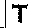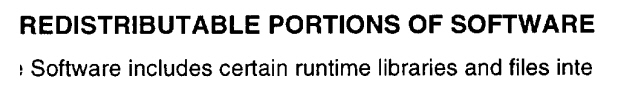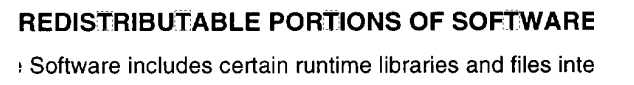﻿ CorrelationCommand Class | Leadtools.ImageProcessing.Core | Raster, Medical, Document Help
←Select platform
In This Topic ▼

# CorrelationCommand Class

Summary

This command compares the correlation image(or part of the correlation image) with all the areas of the same dimensions in the image to be searched (the Run method image) and finds those portions that match according to the measure of correlation. This command is available in the Document/Medical Toolkits.

Syntax
C#
VB
Objective-C
C++
Java
``public class CorrelationCommand : RasterCommand ``
````Public Class CorrelationCommand  `
`   Inherits RasterCommand ````
``@interface LTCorrelationCommand : LTRasterCommand ``
``public class CorrelationCommand extends RasterCommand ``
``public ref class CorrelationCommand : public RasterCommand   ``

Remarks
• This command compares the correlation image (or part of the correlation image) with all the areas of the same dimensions in the image to be searched and finds those portions that match according to the measure of correlation. Correlation is a measure of association (resemblance) between two images. It varies from 0 (zero resemblance) to 100 (perfect resemblance). This command updates the Points property (or the points parameter of the Constructor) with the point of origin for those areas of the image to be searched where the resemblance with the correlation image (or part of the correlation image) is greater than the threshold. The correlation image dimensions must be less than or equal to the dimensions of the image to be searched.
• For example:

1. Select an image of the object for which you want to look. Put it in the CorrelationImage property (or the correlationImage parameter of the constructor). For example:2. Select an image that contains the image you wish to search and put it in the affected image. For example:3. Call CorrelationCommand.

4. The command will update the Points array property (or the points parameter of the Constructor) with the point of origin (top-left) for each area of the image to be searched where the correlation with the correlation image is greater than the correlation threshold. The height and width of the rectangles are the same as for the correlation image.
5. The result is:• This command supports 12 and 16-bit grayscale and 48 and 64-bit color images. Support for 12-bit and 16-bit grayscale and 48-bit and 64-bit color images is available only in the Document/Medical toolkits.

• This command does not support 32-bit grayscale images.
• All images must be signed or unsigned.

Example

Run the CorrelationCommand on an image and applies the correlation filter.

C#
VB
````using Leadtools; `
`using Leadtools.Codecs; `
`using Leadtools.ImageProcessing.Core; `
` `
`public void CorrelationCommandExample() `
`{ `
`   // Load an image `
`   RasterCodecs codecs = new RasterCodecs(); `
`   codecs.ThrowExceptionsOnInvalidImages = true; `
` `
`   RasterImage image = codecs.Load(Path.Combine(LEAD_VARS.ImagesDir, "clean.tif")); `
` `
`   // Prepare the command `
`   RasterImage DstImage = image.Clone(); `
`   CorrelationCommand command = new CorrelationCommand(); `
`   command.CorrelationImage = DstImage; `
`   command.Threshold = 70; `
`   command.XStep = 1; `
`   command.YStep = 1; `
`   command.Points = new LeadPoint; `
`   //Apply the correlation filter. `
`   command.Run(image); `
` `
`} `
` `
`static class LEAD_VARS `
`{ `
`   public const string ImagesDir = @"C:\Users\Public\Documents\LEADTOOLS Images"; `
`} ````
````Imports Leadtools `
`Imports Leadtools.Codecs `
`Imports Leadtools.ImageProcessing.Core `
` `
`Public Sub CorrelationCommandExample() `
`   Dim codecs As New RasterCodecs() `
`   codecs.ThrowExceptionsOnInvalidImages = True `
` `
`   Dim leadImage As RasterImage = codecs.Load(Path.Combine(LEAD_VARS.ImagesDir, "clean.tif")) `
` `
`   ' Prepare the command `
`   Dim points() As LeadPoint `
`   ReDim points(89) `
` `
`   Dim DstImage As RasterImage = leadImage.Clone() `
`   Dim command As CorrelationCommand = New CorrelationCommand `
`   command.CorrelationImage = DstImage `
`   command.Threshold = 70 `
`   command.XStep = 1 `
`   command.YStep = 1 `
`   command.Points = points `
`   'Apply the correlation filter. `
`   command.Run(leadImage) `
` `
`End Sub `
` `
`Public NotInheritable Class LEAD_VARS `
`   Public Const ImagesDir As String = "C:\Users\Public\Documents\LEADTOOLS Images" `
`End Class ````
````c#[Silverlight C# Example] `
`using Leadtools; `
`using Leadtools.Examples; `
`using Leadtools.Codecs; `
`using Leadtools.ImageProcessing.Core; `
` `
`public void CorrelationCommandExample(RasterImage image, Stream outStream) `
`{ `
`   // Prepare the command `
`   RasterImage DstImage = image.Clone(); `
`   CorrelationCommand command = new CorrelationCommand(); `
`   command.CorrelationImage = DstImage; `
`   command.Threshold = 70; `
`   command.XStep = 1; `
`   command.YStep = 1; `
`   command.Points = new LeadPoint; `
`   //Apply the correlation filter. `
`   command.Run(image); `
` `
`   // Save result image `
`   RasterCodecs codecs = new RasterCodecs(); `
`   codecs.Save(image, outStream, RasterImageFormat.Jpeg, 24); `
`   image.Dispose(); `
`} `
`vb[Silverlight VB Example] `
`Imports Leadtools `
`Imports Leadtools.Codecs `
`Imports Leadtools.ImageProcessing.Core `
` `
`Public Sub CorrelationCommandExample(ByVal image As RasterImage, ByVal outStream As Stream) `
`   ' Prepare the command `
`   Dim DstImage As RasterImage = image.Clone() `
`   Dim command As CorrelationCommand = New CorrelationCommand() `
`   command.CorrelationImage = DstImage `
`   command.Threshold = 70 `
`   command.XStep = 1 `
`   command.YStep = 1 `
`   command.Points = New LeadPoint(89) {} `
`   'Apply the correlation filter. `
`   command.Run(image) `
` `
`   ' Save result image `
`   Dim codecs As RasterCodecs = New RasterCodecs() `
`   codecs.Save(image, outStream, RasterImageFormat.Jpeg, 24) `
`   image.Dispose() `
`End Sub ````

Requirements

Help Version 20.0.2020.4.3